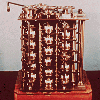#### You may also like### Route to Root

A sequence of numbers x1, x2, x3, ... starts with x1 = 2, and, if you know any term xn, you can find the next term xn+1 using the formula: xn+1 = (xn + 3/xn)/2 . Calculate the first six terms of this sequence. What do you notice? Calculate a few more terms and find the squares of the terms. Can you prove that the special property you notice about this sequence will apply to all the later terms of the sequence? Write down a formula to give an approximation to the cube root of a number and test it for the cube root of 3 and the cube root of 8. How many terms of the sequence do you have to take before you get the cube root of 8 correct to as many decimal places as your calculator will give? What happens when you try this method for fourth roots or fifth roots etc.?### Divided Differences

When in 1821 Charles Babbage invented the `Difference Engine' it was intended to take over the work of making mathematical tables by the techniques described in this article.### Geomlab

A geometry lab crafted in a functional programming language. Ported to Flash from the original java at web.comlab.ox.ac.uk/geomlab

# Odd One Out

### Why do this problem?

This problem helps to develop the skill of working with large sets of numbers and getting a 'feel' for their properties. Being able to spot anomalous data quickly is very useful in industrial and research contexts, and this problem could be considered numerical detective work.

### Possible approach

This activity could be used with small groups of students working on computers or with the whole class working together, perhaps with printed copies of a dataset. Give students plenty of time to study the data and discuss in small groups anything they notice.

When they think they have identified an odd one out for most or all of the six processes, students could explain to one another how they think the processes work and how sure they are that they have correctly identified the odd one out. They could then test their identification of the processes with new data.

### Key questions

Are there any patterns to the data? Is there anything that doesn't fit with the patterns you see?
Can we ever be sure that our explanation of the processes is correct?
At what point do we accept that our explanation is correct?

### Possible extension

There is scope for lots of statistical calculation to justify the decisions students make for the odd ones out, by calculating the probability of those errors arising by chance, and working out how likely it is that the data was generated by processes other than those they have assumed.

### Possible support

The problem Data Matching gives an opportunity to look at and compare data sets.